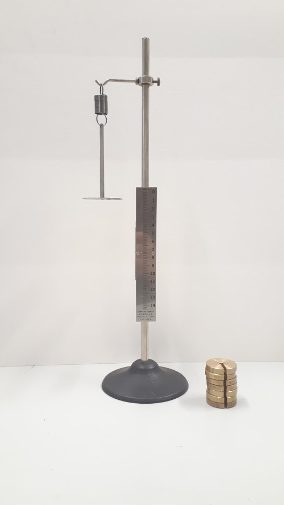# Hookes Law

When a helical spring is stretched from its unextended position the end of the spring exerts a restoring force which is proportional to the magnitude of the extension from equilibrium.  Thus the restoring force when the displacement from equilibrium is x is given by

F = – kx

where k is a constant characteristic of the particular spring involved.  The objective of this experiment is to investigate if the spring provided does indeed obey the above equation (sometimes called Hooke’s law) and, if so, to determine the value of the constant k for that spring.The easiest way to apply a known force to extend a spring is to hang the spring so that its axis is vertical and then to hang known masses from the end of the spring.  The upper end of the spring is hung from a stand while a weight hanger is attached to the lower end.  A pointer attached to the weight hanger can be rotated so that it points to the graduations on a scale which is parallel to the spring.  The height of the scale can be adjusted so that the pointer is initially near the top of the graduated portion, which can be taken as the point of zero extension. To eliminate parallax, the pointer should be viewed when it is exactly in front of its image in the mirrored surface of the scale.

By adding different masses to the weight hanger, different forces can be applied to the spring. The pointer moves downwards along the vertical scale and the extensions corresponding to each applied mass can be recorded.

If you want to explore hookes law yourself then try you hand with these online labs / simulations:

Masses and Springs

Verification of Hookes Law

## Department of Physics

Roinn na Fisice

Room 213 (Physics Office), 2nd floor, Kane Science Building, University College Cork, Ireland.,

Top